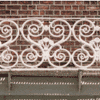#### You may also like### Is There a Theorem?

Draw a square. A second square of the same size slides around the first always maintaining contact and keeping the same orientation. How far does the dot travel?### Khun Phaen Escapes to Freedom

Slide the pieces to move Khun Phaen past all the guards into the position on the right from which he can escape to freedom.### Frieze Patterns in Cast Iron

A gallery of beautiful photos of cast ironwork friezes in Australia with a mathematical discussion of the classification of frieze patterns.

# Translating Lines

##### Age 11 to 14Challenge Level

Move the red dots to set the position of the red line. Then move the blue dot to translate the blue line up and down.

Investigate what happens to the equations of different lines when you translate them up or down.
Try to predict what will happen. Explain your findings.

Now investigate what happens to the equations of different lines when you translate them left or right.
Try to predict what will happen. Explain your findings.

Below are the equations of ten lines, and some translations. Each translation links a pair of parallel lines - can you match them up?

 $y=2x-5$ $y=-2x+6$ Right 4 $y=\frac13x+24$ $y=2x+12$ Right 5, Up 5 $y=-\frac13x-11$ $y=\frac13x+21$ Left 1, Up 2 $y=-2x-9$ $y=2x-9$ Left 6, Down 5 $y=2x+4$ $y=-\frac13x-12$ Left 3

Can you find other combinations of vertical and horizontal translations that link each pair of parallel lines?